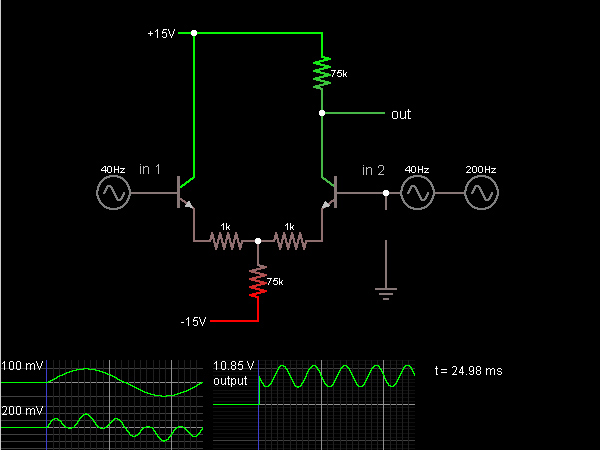# Circuit Simulator - Differential Amplifier (with transistors)

## Where can I get Differential Amplifier (with transistors) Circuit Diagram with Explanation?

IndiaBIX provides you lots of fully solved Differential Amplifier (with transistors) circuit diagram with detailed explanation and working principles.

## How to design a Differential Amplifier (with transistors) (electronic circuit)?

You can easily design the Differential Amplifier (with transistors) circuit by practicing the exercises given below. Here you can design and simulate your own electronic circuits with this Online Circuit Designer and Simulator.### Circuit Description:

This is a differential amplifier built using two transistors. The output is equal to the voltage difference between the two inputs. Input 1 is a 40Hz signal, and input 2 is a 40Hz signal with a 200 Hz signal added in. (In this simulation, the two signals are added simply by connecting the two sources in series, which is convenient but not realistic.) The output is the 200Hz signal.

The two inputs are connected to the bases of the two transistors. The emitter of each is a diode drop lower than the base.

In the differential-mode case, when input 1 rises and input 2 falls by the same amount, there is more voltage across the left 1k resistor, and so more current. There is less voltage/current across the right 1k. It adds up to the same voltage and current through the 75k. The reduced current through the right transistor causes the output to rise. When input 1 falls and input 2 rises, the output falls.

In the common-mode case, when input 1 and input 2 rise together, that means more voltage/current across both 1k's, which means more voltage/current across the 75k as well. But the 75k is a large resistor, and so a small increase in current produces a large increase in voltage. So the change in current through the right transistor (and the voltage change in output) is much less in common mode than in differential mode. -- Credits: Mr. Paul Falstad.